## Calculating Value at Risk using R

### Tags: Big Data, Damian Spendel, Financial Services, Innovation, R, Value, VaR

#### 1

Introduction

My recent article focused on using R to perform some basic exploratory data analysis1.

The focus of this article will be to highlight some packages that focus on financial analytics (TTR, quantmod and PerformanceAnalytics) and a package that will allow us to build an interactive UI with a package called Shiny.

For this article we will focus on Value at Risk2, a common market risk measure developed by JP Morgan and most recently criticized by Nassim Taleb3.

Historical Simulation – Methodology

For the first part of this article I will walk through the methodology of calculating VaR for a single stock using the historical simulation method (as opposed to the Monte Carlo or parametric method)4.

VaR allows a risk manager to make a statement about a maximum loss over a specified horizon at a certain confidence level.

V will be the Value at Risk for a one day horizon at a 95% confidence level.

Briefly, this method is: retrieve and sort a returns timeseries from a specified period (usually 501 days) and take a specific quantile and you will have the Value at Risk for that position.

Note however this will only apply to a single stock, I will cover multiple stocks in a later article. Normally a portfolio will not only include multiple stocks, but forwards, futures and other derivative positions.

In R, we would proceed as follows.

 ##pre-requisite packages 
 library(quantmod) 
 library(PerformanceAnalytics)

With the packages loaded we can now run through the algorithm:

 X <- c(0.95) 
stock <- c("AA") ##American Airlines 
## define the historical timeseries 
begin <- Sys.Date() - 501 
end <- Sys.Date() 
## first use of quantmod to get the ticker and populate our dataset with 
the timeseries of Adjusted closing price 
tickers <- getSymbols(stock, from = begin, to = end, auto.assign = TRUE) 
dataset <- Ad(get(tickers)) 
## now we need to convert the closing prices into a daily returns 
timeseries - we will use the Performance Analytics package 
returns_AA <- Return.calculate(dataset, method=c("simple"))

We now have the dataset and can start to do some elementary plotting, firstly the returns timeseries to have a quick look:

 chartSeries(returns_AA)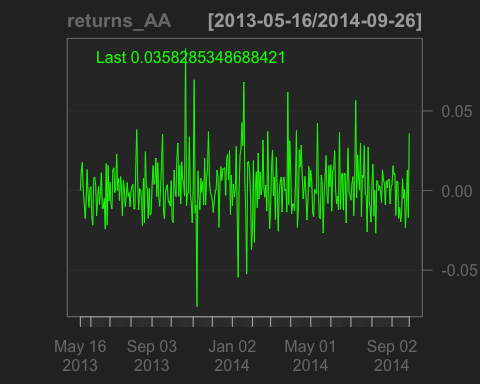Now, we’ll convert the timeseries into a sorted list and apply the quantile function

 ##convert to matrix datatype as zoo datatypes can't be sorted, then sort ascending 
returns_AA.m <- as.matrix(returns_AA); sorted <- 
returns_AA.m[order(returns_AA.m[,1])] 
##calculate the 5th percentile, 
##na.rm=TRUE tells the function to ignore NA values (not available values) 
100*round(quantile(returns_AA.m[order(returns_AA.m[,1])], c(1-X), na.rm=TRUE), 4) 
## 5% 
## -2.14

This shows us that the 5% one day value at risk for a position in American Airlines is -2.14%, that is, for $100 of position, once every 20 days you would lose more than$2.14.

Building a UI

A worthwhile guide to using Shiny is available on the Shiny Website. (http://shiny.rstudio.com/tutorial/)

In essence, we will need to define two files in one directory, server.R and UI.R.

We’ll start with the UI code, not that I have used the “Telephones by Region” as a template (http://shiny.rstudio.com/gallery/telephones-by-region.html).

The basic requirements are:

1. A drop-down box to choose the stock.
2. A function that plots a histogram of the returns time-series and shows the VaR as a quantile on the histogram.
##get the dataset for the drop-down box, 
##we'll use the TTR package for downloading a vector of stocks, 
##and load this into the variable SYMs 
library(TTR) 
library(sqldf) 
library(shiny) 
suppressWarnings(SYMs <- TTR::stockSymbols()) 
##use the handy sqldf package to query dataframes using SQL syntax
##we'll focus on Banking stocks on the NYSE. 
SYMs <- sqldf("select Symbol from SYMs where Exchange='NYSE' and Industry like '%Banks%'") 
# Define the overall UI, shamelessly stolen from the shiny gallery 
shinyUI( 
 # Use a fluid Bootstrap layout 
 fluidPage( 
 # Give the page a title 
 titlePanel("NYSE Banking Stocks - VaR Calculator"), 
 # Generate a row with a sidebar, calling the sidebar "Instrument" and populating the choices with the vector SYMs 
 sidebarLayout( selectInput("Instrument", "Instrument:", choices=SYMs), 
 hr(), 
 ), 
# Create a spot for the histogram 
 mainPanel(plotOutput("VaRPlot"))
 ) 
)

With the UI layout defined, we can now define the functions in the Server.R code:

shinyServer(function(input, output){ 
# Fill in the spot we created in UI.R using the code under "renderPlot" 
 output$VaRPlot<-renderPlot({   ##use the code shown above to get the data for the chosen instrument captured in input$Instrument 
 begin <- Sys.Date() - 501 
 end <- Sys.Date() 
 tickers <- getSymbols(input$Instrument, from = begin, to = end,   auto.assign = TRUE)   dataset <- Ad(get(tickers))   dataset <- dataset[,1]  returns <- Return.calculate(dataset, method=c("simple"))   ##use the quantmod package that creates the histogram and adds 95% VaR using the add.risk method   chart.Histogram(returns, methods = c("add.risk"))   })  }) In RStudio, you will then see the button “Run App”, which after clicking will run your new and Shiny app.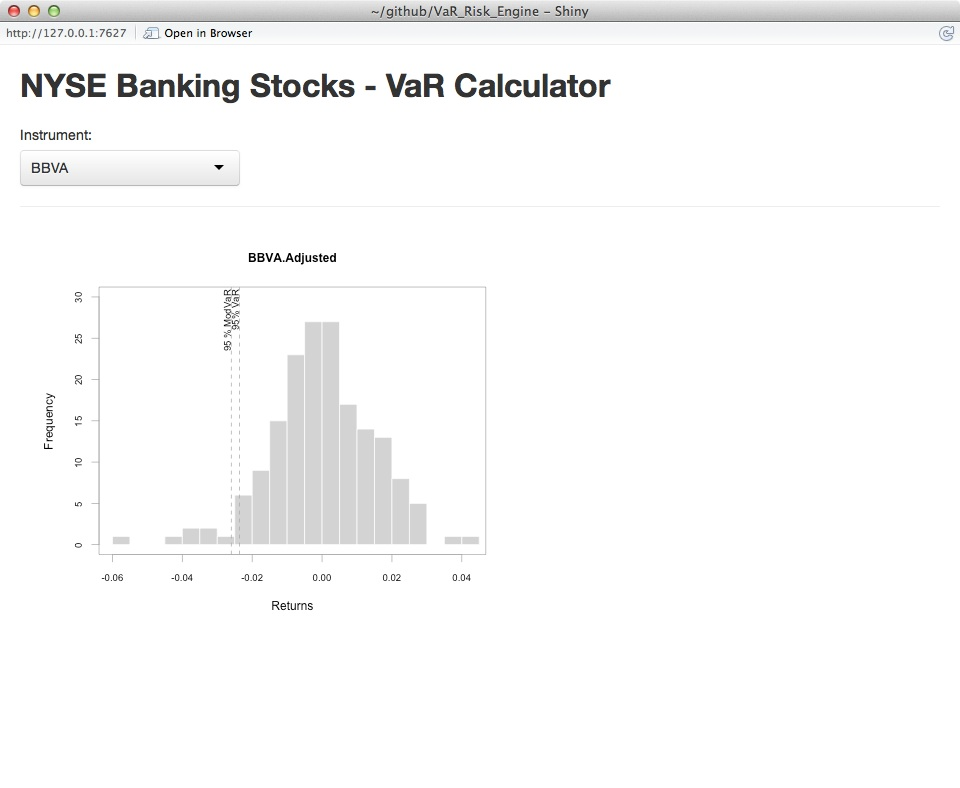Guest author: Damian Spendel – Damian has spent his professional life bringing value to organisations with new technology. He is currently working for a global bank helping them implement big data technologies. You can contact Damian at damian.spendel@gmail.com ## Data Analysis – An example using R ### Posted on : 31-08-2014 | By : richard.gale | In : Data ### Tags: Big Data, Complexity, Data Science, Data Visualisation, programming, R, Technology #### 3 With the growth of Big Data and Big Data Analytics the programming language R has become a staple tool for data analysis. Based on modular packages (4000 are available), it offers sophisticated statistical analysis and visualization capabilities. It is well supported by a strong user community. It is also Open Source. For the current article I am assuming an installation of R 3.1.1 and RStudio. The article will cover the steps taken to provide a simple analysis of highly structured data using R. The dataset I will use in this brief demonstration of some basic R capabilities is from a peer-to-peer Lending Club in the United States. The dataset is well structured with a data dictionary and covers both loans made and loans rejected. I will use R to try and find answers to the following questions: • Is there a relationship between Loan amounts and funded amounts? • Is there a relationship between the above and the number of previous public bankruptcies? • What can we find out about rejections? • What can we find out about the geographic distribution of the Lending Club loans? During the course of this analysis we will use basic commands R to: • Import data • Plot data using scatterplots and regression lines • Use functions to perform heavy lifting • Summarize data using simple aggregation functions • Plot data using choropleths Having downloaded the data to our working directory we’ll import the three files using read.csv and merge them together using rbind() (row bind): >data_lending0 <- read.csv(“LoanStats3a.csv”, header =FALSE) >data_lending1 <- read.csv(“LoanStats3b.csv”, header =FALSE) >data_lending2 <- read.csv(“LoanStats3c.csv”, header =FALSE) >data_full <- rbind(data_lending0, data_lending1, data_lending2) We can now explore the data using some of R’s in-built functions for metadata – str (structure), names (column names), unique (unique values of a variable). The first thing I will do is use ggplot to build a simple scatter plot showing the relationship between the funded amount and the loan amount. >install.packages(“ggplot2″) >library(ggplot2) >ggplot(data_full, aes(x=loan_amnt, y=funded_amnt)) + geom_point(shape=1) + geom_smooth(method=lm) The above three lines install the package ggplot2 from a CRAN mirror, load the library into the R environment, and then use the “Grammar of Graphics” to build a plot using the data_full dataset, with the x-axis showing the principle (loan_amnt) and the y-axis showing the lender’s contribution (funded_amnt). With geom_smooth we add a line to help see patterns – in this case a line of best fit. In R Studio we’ll now see the following plot: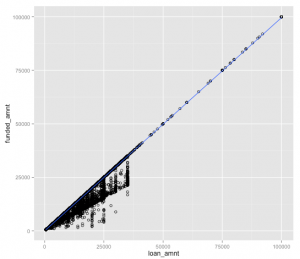This shows us clearly that the Lending Club clusters loans at the lower end of the spectrum and that there is a clear positive correlation between the loan_amount and funded_amnt – for every dollar you bring you can borrow a dollar, there is little scope for leverage here. Other ggplot functions will allow us to tidy up the labeling and colours, but I’ll leave that as an exercise for the interested reader. The next step is to add an additional dimension – and investigate the link between principles and contributions under the aspect of known public bankruptcies of the applicants. >ggplot(data_full, aes(x=loan_amnt, y=funded_amnt, color=pub_rec_bankruptcies)) + geom_point(shape=19,alpha=0.25) + geom_smooth(method=lm) Here, I’ve used the color element to add the additional dimension and attempted to improve legibility of the visualization by making the points more transparent.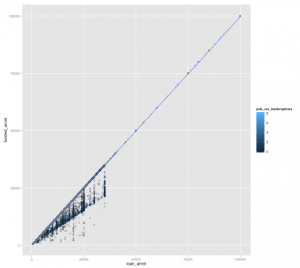Not very successfully, it doesn’t help us further – maybe sampling could improve the visualization, or a more focused view…. Let’s have a quick look at the rejection statistics: > data1 <- rbind(read.csv(“RejectStatsA.csv”), read.csv(“RejectStatsB.csv”)) > nrow(rejections)/nrow(data_full)  6.077912 For every application – six rejections. Another popular method of visualization is using choropleth (“many places”) visualizations. In this case, we’ll build a map showing outstanding loans by State. The bad news is that the Lending Club data uses two-letter codes and that state data we’ll use from the maps package (install.packages, library etc….) is the full name. Fortunately, a quick search provides a function “stateFromLower” that will perform this for us. So, I run the code that creates the function and then add a new column called state (“WY”) to the data_full dataset, and use stateFromLower to conver the addr_state column (“Wyoming”): > data_full$state <- stateFromLower(data_lending$addr_state Then, I aggregate the principles by state: > loan_amnts <- aggregate(data_lending$funded_amnt, by=list(data_lending$state), FUN=sum) Load the state data: > data(state) The next code leans heavily on a succinct tutorial provided elsewhere: > map(“state”, fill=FALSE, boundary=TRUE, col=”red”) > mapnames <- map(“state”, plot=FALSE)$names

Remove regions:

> region_list <- strsplit(mapnames, “:”)

> mapnames2 <- sapply(region_list, “[“, 1)

Match the region-less mapnames to the loan amounts:

> m <- match(mapnames2, tolower(state.name))

> loans <- loan_amnts$x Bucketize the aggregated loans: > loan.buckets <- cut(loans, c(“500000”, “1000000”, “5000000”, “10000000”, “15000000”, “20000000”, “30000000”, “40000000”, “90000000”, “100000000”)) Define the colour schema: > clr <- rev(heat.colors(13)) Draw the map and add the title: > map(“state”, fill=TRUE, col=clr[loan.buckets], projection = “polyconic”) > title(“Lending Club Loan Amounts by State”) Create a legend: > leg.txt <- c(“$500000”, “$1000000”, “$5000000”, “$10000000”, “$15000000”, “$20000000”, “$30000000”, “$40000000”, “$90000000”, “\$100000000”)

> legend(“topright”, leg.txt, horiz = FALSE, fill = clr)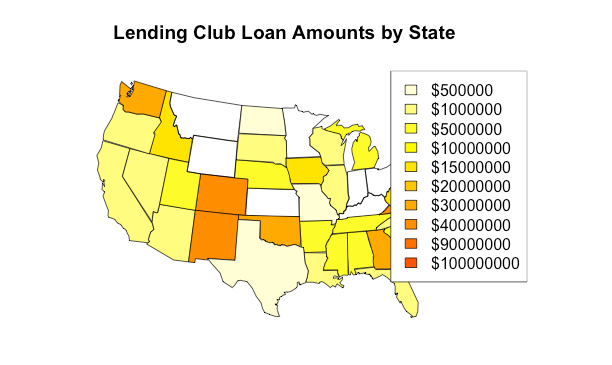With a few simple lines of code R has demonstrated that it is quite a powerful tool for generating visualizations that can aid in understanding  and analyzing data.    We were able to understand something about the lending club – it almost seems like we have clear KPIs in terms of rejections and in terms of loan amounts to funding amounts.    I think we can also see a link between the Lending Club’s presence and poverty.

This could be a starting point for a more detailed analysis into the Lending Club, to support investing decisions, or internal business decisions (reduce rejections, move into Wyoming etc.).

Guest author: Damian Spendel – Damian has spent his professional life bringing value to organisations with new technology. He is currently working for a global bank helping them implement big data technologies. You can contact Damian at damian.spendel@gmail.com